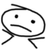# Converting Field Calculator Pre-Logic Script to pyscript

3329
7
07-01-2016 03:41 AMOccasional Contributor

I've been struggling to emulate a simple Field Calculator function as pyscript. When I try, I get parsing errors.

For example, how should this simple function (when manually applied in the Field Calculator tool) look when written up as pyscript?

In Pre-Logic Script Code:

def LookUp ( a, b ) :

if ( a == 0 ) :

newval = 0

else :

newval = b

return newval

In FIELD =

LookUp ( !MAX_RANK!, !Shape_Area! )

Any advice will be much appreciated.

Tags (1)
1 Solution

Accepted SolutionsOccasional Contributor

Code I used:

# Populate SUBJECT_AREA only if there is a Rank shown in MAX_RANK field.

expression = "LookUp(!MAX_RANK!, !Shape_Area!)"

codeblock = "def LookUp(MAX_RANK, Shape_Area):\n\

if MAX_RANK == 0:\n\

return 0\n\

else:\n\

return Shape_Area"

gp.CalculateField_management(currentWorkspace + "/myDataset", "SUBJECT_AREA", expression, "PYTHON", codeblock)

7 RepliesbyMVP Esteemed Contributor

Try this... if there are any errors it is because I can't test it from an iThingy since you can't run Arc* stuff on them

You basically want an update cursor

UpdateCursor—Help | ArcGIS for Desktop

```import arcpy
fc = "c:/path_to/Your_gdb/my.gdb/yourlayer"
field1 = "Shape_Area"
field2 = "MAX_RANK"
calc_fld = "Calculate_field"
cursor = arcpy.UpdateCursor(fc)
row = cursor.next()
while row:
# field2 will be equal to field1 multiplied by 3.0
if row.getValue(field1) == 0.0:
row.setValue(0.0)
else:
row.setValue(calc_fld, row.getValue(field1))
cursor.updateRow(row)
row = cursor.next()```Occasional Contributor

Thanks Dan.

fc = currentWorkspace + "/dataset"

field1 = "MAX_RANK"

field2 = "Shape_Area"

calc_fld = "Calculate_field"

cursor = arcpy.UpdateCursor(fc)

row = cursor.next()

while row:

if row.getValue(field1) == 0:

row.setValue(0)

else:

row.setValue(calc_fld, row.getValue(field2))

cursor.updateRow(row)

row = cursor.next()

Runtime error

Traceback (most recent call last):

File "<string>", line 35, in <module>

File "c:\program files (x86)\arcgis\desktop10.1\arcpy\arcpy\arcobjects\arcobjects.py", line 1040, in setValue

return convertArcObjectToPythonObject(self._arc_object.SetValue(*gp_fixargs(args)))

AttributeError: Row: Error in parsing arguments for SetValue

I'll keep chipping away at it.Regular Contributor III

The simplest way to get the proper syntax is to use your calculation in model builder then export as python script.byMVP Esteemed Contributor

Wes... which assumes the model works first go... but based on the number of model failures on this site, that would be my last choiceOccasional Contributor

Thanks Wes. I tried this and it returned:

Failed to execute. Parameters are not valid.

ERROR 000989: Python syntax error: Parsing error SyntaxError: invalid syntax (line 1)

Failed to execute (CalculateField).Regular Contributor III

Could you post a screen shot of the model builder field calculator.Occasional Contributor

Code I used:

# Populate SUBJECT_AREA only if there is a Rank shown in MAX_RANK field.

expression = "LookUp(!MAX_RANK!, !Shape_Area!)"

codeblock = "def LookUp(MAX_RANK, Shape_Area):\n\

if MAX_RANK == 0:\n\

return 0\n\

else:\n\

return Shape_Area"

gp.CalculateField_management(currentWorkspace + "/myDataset", "SUBJECT_AREA", expression, "PYTHON", codeblock)